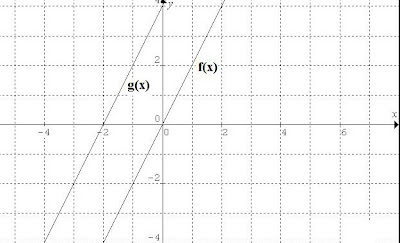## Monday, November 15, 2010

### Those math solutions!In my earlier blog, I gave a sample test that most U.S. High schoolers are unable to do. The blog was about the poor performance of American math students vs. those of other nations, indicating the USA placed 31st among 56 entries. For those who attempted the problems, the solutions are given below:

Simplify 1-3 as much as possible:

1)5(2x^2 - 6x)- (4x^2 - 3x)

Expand both quantities using the distributive law:

-> 10x^2 - 30x - 4x^2 + 3x

Combine terms:

= 6x^2 - 27 x

Simplify via factoring: 6x^2 - 27 x = 3x(2x - 9)

2) 4^1/2 - 3^1/2

= 4 { ^1/2 ^1/2} - 3 {^1/2 ^1/2}

= 4 (5) ^1/2 - 3(3)^1/2 = 20^1/2 - 9^1/2 = 11^1/2

3) (x^2 + 2x - 3)/ (x^2 - 3x + 2)

We approach this by seeing if we can factor the numerator and denominator separately:

Numerator: (x^2 + 2x - 3) = (x + 3) (x - 1)

Denominator: (x^2 - 3x + 2) = (x - 2) (x - 1)

The put back in the factored form:

(x + 3)(x - 1) / (x - 2) (x - 1)

from which we immediately see that the factor (x - 1) cancels from the numerator and the denominator, leaving:

(x + 3)/ ( x- 2)

In 4-5, factor completely:

4) x^2 - 9x + 18

= (x - 3) (x - 6)

5) x^2 + 10x + 25 - 9y^2

= (x + 5) (x + 5) - 3y(3y)

6) Graph the functions: f(x) = 2x and g(x) = 2x + 4 on the same coordinate axes

This is easy and the solution is shown on the graph above. Basically, one selects a series of x-values (e.g. x = -2, -1, 0, 1, 2, 3, 4 ..) and obains f(x) and g(x) from the operation, then plots the coordinate pairs resulting.

7) Find the slope of any line that is perpendicular to the line whose equation is:

x + 4y - 8 = 0

Best approach is write in point-slope form: y = mx + c

so the equation is rewritten: 4y = -x + 8 or y = - ¼ x + 2

Now, any line (equation) perpendicular to the above, must have the negative reciprocal of the slope (m). Recall the reciprocal is that number which when multiplied by the original number yields 1. Since m = - ¼, then the negative reciprocal of it is 4. (E.g. the ordinary reciprocal is: 1/(- ¼) = -4 and its negative is 4.

8) Write the equation of the line passing through the point (3, -5) and perpendicular to the line whose equation is: x + 4y - 8 = 0

We use the point slope form again, in the more general format:

(y - y1) = m(x - x1)

where (x1, y1) = (3, -5)

Then: y - (-5) = m(x - 3)

We note the equation of interest, x + 4y - 8 = 0, is the same one in (7). Thus, we know already that the slope must be m = 4 (the negative reciprocal of - ¼). Therefore:

y + 5 = 4(x - 3) or y = 4x -12 -5

The equation of the line is: y = 4x - 17

9) Solve the quadratic equation:x^2 - 4x + 16 = 0

Cursory inspection shows this cannot be solved by factoring, so we must resort to the quadratic formula:

x(1,2) = {- b +/- [b^2 - 4 ac]^1/2 } / 2a

where x(1,2) denotes the roots, x1 and x2

And we define the coefficients: a =1, b = -4, c = 16

working this out, we get:

x(1,2) = {- (-4) +/- [(-4)^2 - 4 (1)(16)]^1/2 } / 2

So: x1 = 2 + 3.464i

x2 = 2 - 3.464i

10) A student fires a model rocket in a field and wishes to obtain its altitude. He has his friend stand exactly 300 meters away with a theodolite that measures the angle of the rocket at its maximum altitude. If the angle is found to be 60 degrees, how high did the rocket go?

The first thing here is to set up the applicable geometry which is shown in the accompanying diagram.

From this we see we must use the tangent trigonometric relation- which is defined in terms of:

tangent (Angle) = side opposite/ side adjacent

In this case, the side opposite = y, the maximum altitude, and x = the distance of the measuring device (theodolite) from the rocket launch site, = 300m.

Then:

tan (60) = y / x

and y = tan (60) x

so y = 1.732 (300 m) = 519 m

Hopefully, any high school blog readers got all correct!